Search IntMath
Close

450+ Math Lessons written by Math Professors and Teachers

5 Million+ Students Helped Each Year

1200+ Articles Written by Math Educators and Enthusiasts

Simplifying and Teaching Math for Over 23 Years

# Mathematica Player and arty Demonstrations

By Murray Bourne, 31 Mar 2009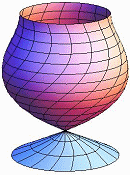The Mathematica Player is a free download. It allows the user to interact with math objects made using the Mathematica computer algebra system.

There is an extraordinary collection of such Mathematica objects — almost 5000 of them — in the Wolfram Demonstrations Project.

This download is really cool because you get a working Mathematica math engine. You can't create anything or save anything but you can play with all the math objects (by sliding sliders or otherwise changing numerical parameters) to explore a vast range of math and geometry. They give you an animation of each one, but it is much better to get the download since you can change the parameters however you like and you learn a lot more.

The Demonstrations have been contributed by mathematicians all over the world. They cover the following topics:

• Mathematics
• Computation
• Physical Sciences
• Life Sciences
• Systems, Models, & Methods
• Engineering & Technology
• Our World
• Creative Arts
• Kids & Fun

The following screen shots are from some of the demonstrations. I liked these ones for their artistic geometry.

This one is called "Line Art":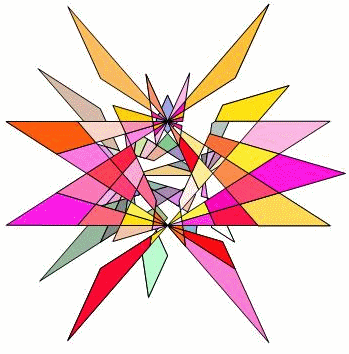Next we have a 3-D demonstration of parabolic cylindrical coordinates: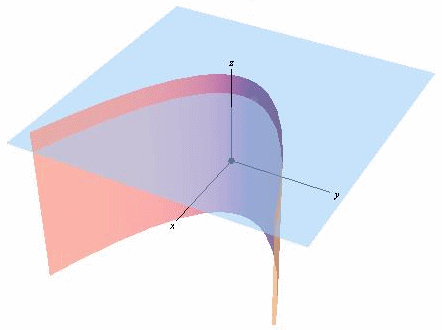This is a circle formed from intersecting chords of a larger circle:This next one is called "Connected Astroids". For those of you (like me) who are not sure what an astroid is, from Wikipedia:

An astroid is a hypocycloid with four cusps. Astroids are also superellipses: all astroids are scaled versions of the curve specified by the equation x2/3 + y2/3 = 1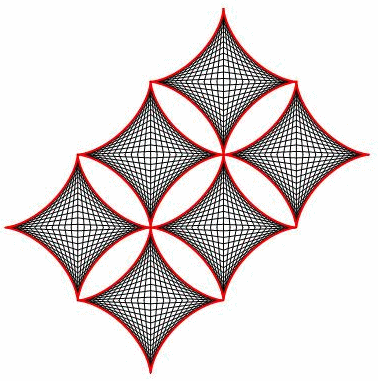Real Elliptic Curves arise from the intersection of a curve and a plane: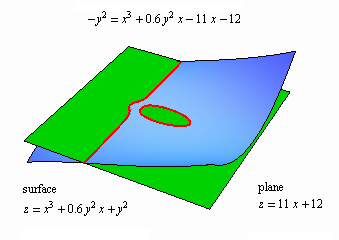So go download the Mathematica Player and start exploring all those thousands of Demonstrations. It's certainly worth it!

### 2 Comments on “Mathematica Player and arty Demonstrations”

1. kewani says:

thank you!!!!!!!!!!!!!

2. Nema Mansuri says:

Nifty.

### Comment Preview

HTML: You can use simple tags like <b>, <a href="...">, etc.

To enter math, you can can either:

1. Use simple calculator-like input in the following format (surround your math in backticks, or qq on tablet or phone):
a^2 = sqrt(b^2 + c^2)
(See more on ASCIIMath syntax); or
2. Use simple LaTeX in the following format. Surround your math with $$ and $$.
$$\int g dx = \sqrt{\frac{a}{b}}$$
(This is standard simple LaTeX.)

NOTE: You can mix both types of math entry in your comment.